Try NerdPal! Our new app on iOS and Android

# Find the derivative of $x<y<0$ using the definition

## Related Videos

Go!
Go!
1
2
3
4
5
6
7
8
9
0
a
b
c
d
f
g
m
n
u
v
w
x
y
z
.
(◻)
+
-
×
◻/◻
/
÷
2

e
π
ln
log
log
lim
d/dx
Dx
|◻|
θ
=
>
<
>=
<=
sin
cos
tan
cot
sec
csc

asin
acos
atan
acot
asec
acsc

sinh
cosh
tanh
coth
sech
csch

asinh
acosh
atanh
acoth
asech
acsch

### Videos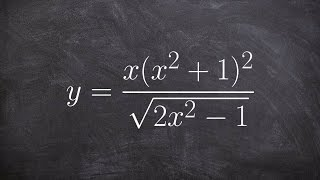### Calculus - Take the log of both sides to find the derivative, y = (x(x^2 + 1)^2)/(sqrt(2x^2 - 1))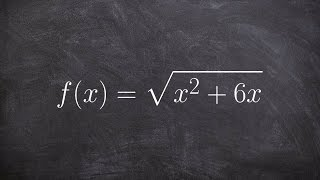### Calculus - Find the derivative of the inverse of a radical equation, f(x)=sqrt(x^2 +6x); find g'(4)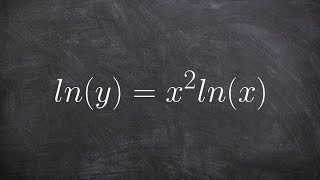### Calculus - Take the derivative using product rule with natural logarithms,, ln(y) = (x^2)ln(x)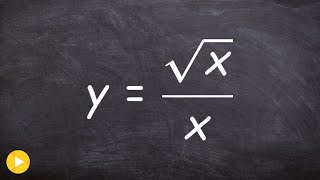### Finding the derivative square root x over x, using power rule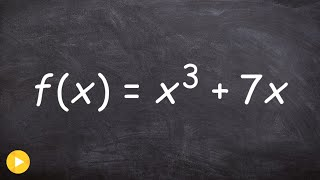### Calculus - Derivative of inverse function given an equation, f(x) = x^3 + 7x; Find g'(8)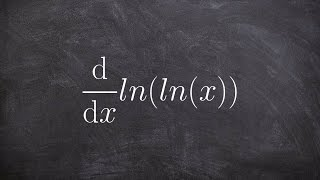### Calculus - Find the double derivative of the natural logarithm, y = ln(lnx); Find y''

$derivdef\left(x<y<0\right)$